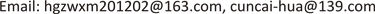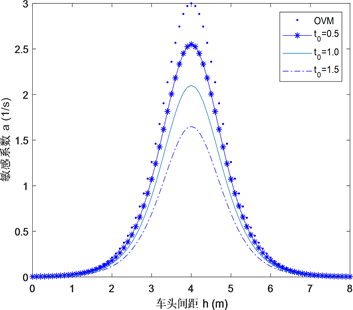﻿ 考虑限速的最优速度模型的稳定性与孤波 The Stability and Soliton of the Optimal Velocity Model Considering Speed Limit

International Journal of Mechanics Research
Vol. 08  No. 03 ( 2019 ), Article ID: 32084 , 10 pages
10.12677/IJM.2019.83021

The Stability and Soliton of the Optimal Velocity Model Considering Speed Limit

Guangzhu He, Cuncai Hua

School of Mathematics, Yunnan Normal University, Kunming YunnanReceived: Aug. 19th, 2019; accepted: Sep. 2nd, 2019; published: Sep. 9th, 2019ABSTRACT

Based on the optimal velocity model, this paper designs a traffic flow model which takes into account the driver’s advance time to know the speed limit information. By using the linear stability analysis method, the stability condition of the model is obtained. It shows that the influence of the speed limit makes the stable region of traffic flow expand obviously. The density wave equations such as Burgers equation, KdV equation and mKdV equation are derived respectively from the reduced perturbation method in the stable region, metastable region and unstable region. The phenomena of traffic congestion under the speed limit are described by the solitary wave solution of Burgers and KdV equation, and by the kink-antikink solution of mKdV equation.

Keywords:The Optimal Velocity Model, Speed Limit, Stability, Density WaveCopyright © 2019 by author(s) and Hans Publishers Inc.1. 引言

2. 模型的提出

1995年，Bando等  提出的最优速度模型很好地描述了交通流的一些非线性现象，其运动方程如下：

${\stackrel{¨}{x}}_{n}\left(t\right)=a\left[V\left(\Delta {x}_{n}\left(t\right)\right)-{v}_{n}\left(t\right)\right]$ (1)

$V\left(\Delta {x}_{n}\left(t\right)\right)=\frac{{v}_{\mathrm{max}}}{2}\left[\mathrm{tanh}\left(\Delta {x}_{n}\left(t\right)-{h}_{c}\right)+\mathrm{tanh}\left({h}_{c}\right)\right]$ (2)

2015年，Lie等  通过调整车辆自身的速度差(当前速度与历史速度)达到稳定交通流的目的，提出了如下自稳定控制驾驶的跟驰模型：

${\stackrel{¨}{x}}_{n}\left(t\right)=a\left[V\left(\Delta {x}_{n}\left(t\right)\right)-{v}_{n}\left(t\right)\right]+\rho \left[{v}_{n}\left(t\right)-{v}_{n}\left(t-{t}_{1}\right)\right]$ (3)

2017年，Chen等  考虑驾驶员对自身车速变化的连续记忆效应，将模型(2)拓展为具有连续记忆效应的如下交通流跟驰模型：

${\stackrel{¨}{x}}_{n}\left(t\right)=a\left[V\left(\Delta {x}_{n}\left(t\right)\right)-{v}_{n}\left(t\right)\right]+\gamma \left[{v}_{n}\left(t\right)-\frac{1}{{t}_{1}}\underset{t-{t}_{1}}{\overset{t}{\int }}{v}_{n}\left(t\right)\text{d}t\right]$ (4)

2018年，Sun等  将车头时距自稳控制因子加入OVM中，提出如下扩展的自稳定最优速度模型：

${\stackrel{¨}{x}}_{n}\left(t\right)=a\left[V\left(\Delta {x}_{n}+\kappa \left(h-\Delta {x}_{n}\right)\right)-{v}_{n}\right]$ (5)

${\stackrel{¨}{x}}_{n}\left(t\right)=a\left[V\left(\Delta {x}_{n}\left(t\right)\right)-{v}_{n}\left(t\right)\right]+\lambda \left[{v}_{n}\left(t+{t}_{0}\right)-{v}_{n}\left(t\right)\right]$ (6)

${\stackrel{¨}{x}}_{n}\left(t\right)=a\left[V\left(\Delta {x}_{n}\left(t\right)\right)-{\stackrel{˙}{x}}_{n}\left(t\right)\right]+\lambda \left[{\stackrel{˙}{x}}_{n}\left(t+{t}_{0}\right)-{\stackrel{˙}{x}}_{n}\left(t\right)\right]$ (7)

3. 模型(7)的稳定性分析

${x}_{n}^{0}\left(t\right)=hn+V\left(h\right)t,h=L/N$ (8)

${x}_{n}\left(t\right)={x}_{n}^{0}\left(t\right)+{y}_{n}\left(t\right)$ (9)

${x}_{n}\left(t\right)$ 为扰动影响下车辆的实际位移。

${\stackrel{¨}{y}}_{n}\left(t\right)=a\left[V\left(h+\Delta {y}_{n}\left(t\right)\right)-V\left(h\right)-{\stackrel{˙}{y}}_{n}\left(t\right)\right]+\lambda \left[{\stackrel{˙}{y}}_{n}\left(t+{t}_{0}\right)-{\stackrel{˙}{y}}_{n}\left(t\right)\right]$ (10)

${\stackrel{¨}{y}}_{n}\left(t\right)=a\left[{V}^{\prime }\left(h\right)\Delta {y}_{n}\left(t\right)-{\stackrel{˙}{y}}_{n}\left(t\right)\right]+\lambda \left[{\stackrel{˙}{y}}_{n}\left(t+{t}_{0}\right)-{\stackrel{˙}{y}}_{n}\left(t\right)\right]$ (11)

${z}^{2}=a\left[{V}^{\prime }\left(h\right)\left(\mathrm{exp}\left(ik\right)-1\right)-z\right]+\lambda z\left({\text{e}}^{{t}_{0}z}-1\right)$ (12)

${z}_{1}={V}^{\prime }\left(h\right)$${z}_{2}=\frac{{V}^{\prime }\left(h\right)}{2}+\frac{\left(\lambda {t}_{0}-1\right){V}^{\prime }{\left(h\right)}^{2}}{a}$ (13)

$a=2\left(1-\lambda {t}_{0}\right){V}^{\prime }\left(h\right)$ (14)(a)(b)

Figure 1. The neutral stability lines in the headway-sensitivity space for different parameter combinations. (a) ${t}_{0}=1$ , (b) $\lambda =0.3$

4. 模型(7)的约化摄动分析和孤立波

$\begin{array}{c}\frac{{\text{d}}^{2}\left(\Delta {x}_{n}\left(t\right)\right)}{\text{d}{t}^{2}}=a\left[V\left(\Delta {x}_{n+1}\left(t\right)\right)-V\left(\Delta {x}_{n}\left(t\right)\right)-\frac{\text{d}\left(\Delta {x}_{n}\left(t\right)\right)}{\text{d}t}\right]\\ \text{\hspace{0.17em}}\text{\hspace{0.17em}}+\lambda \left[\frac{\text{d}\left(\Delta {x}_{n}\left(t+{t}_{0}\right)\right)}{\text{d}t}-\frac{\text{d}\left(\Delta {x}_{n}\left(t\right)\right)}{\text{d}t}\right]\end{array}$ (15)

4.1. 在稳定流区域导出Burgers方程

$X=\epsilon \left(n+bt\right)$$T={\epsilon }^{2}t$ (16)

$\Delta {x}_{n}\left(t\right)=h+\epsilon R\left(X,T\right)$ (17)

$a{\epsilon }^{2}\left(b-{V}^{\prime }\left(h\right)\right){\partial }_{X}R+{\epsilon }^{3}\left[\left({b}^{2}-\frac{a{V}^{\prime }\left(h\right)}{2}-\lambda {t}_{0}{b}^{2}\right){\partial }_{X}^{2}R-a{V}^{″}\left(h\right)R{\partial }_{X}R+a{\partial }_{T}R\right]=0$ (18)

${\partial }_{T}R=\frac{\partial R}{\partial T}$${\partial }_{X}R=\frac{\partial R}{\partial X}$${\partial }_{X}^{k}R=\frac{{\partial }^{k}R}{\partial {X}^{k}}$

$b={V}^{\prime }\left(h\right)$，消去 $\epsilon$ 的二次项后，将(18)简化为：

$a{\partial }_{T}R-a{V}^{″}\left(h\right)R{\partial }_{X}R+{V}^{\prime }\left(h\right)\left({V}^{\prime }\left(h\right)-\frac{a}{2}-\lambda {t}_{0}{V}^{\prime }\left(h\right)\right){\partial }_{X}^{2}R=0$ (19)

$\frac{1}{2}{V}^{\prime }\left(h\right)\left[a-2\left(1-\lambda {t}_{0}\right){V}^{\prime }\left(h\right)\right]>0$ (20)

$\begin{array}{c}R\left(X,T\right)=\frac{1}{|{V}^{″}\left({h}_{c}\right)|T}\left[X-\frac{1}{2}\left({\eta }_{n}+{\eta }_{n+1}\right)\right]-\frac{1}{2|{V}^{″}\left({h}_{c}\right)|T}\left({\eta }_{n+1}-{\eta }_{n}\right)\\ \text{\hspace{0.17em}}\text{\hspace{0.17em}}×\mathrm{tanh}\left[\frac{{c}_{1}}{4|{V}^{″}\left({h}_{c}\right)|T}\left({\eta }_{n+1}-{\eta }_{n}\right)\left(X-{\zeta }_{n}\right)\right]\end{array}$ (21)

4.2. 在不稳定区域导出mKdV方程

$X=\epsilon \left(n+bt\right)$$T={\epsilon }^{3}t$(22)

$\Delta {x}_{n}\left(t\right)={h}_{c}+\epsilon R\left(X,T\right)$ (23)

$\begin{array}{l}a{\epsilon }^{2}\left(b-{V}^{\prime }\right){\partial }_{X}R+{\epsilon }^{3}\left[\left({b}^{2}-\frac{a{V}^{\prime }}{2}-\lambda {t}_{0}{b}^{2}\right){\partial }_{X}^{2}R-{V}^{″}{\partial }_{X}{R}^{2}\right]\\ \text{ }+{\epsilon }^{4}\left[a{\partial }_{T}R-\frac{a{V}^{‴}}{2}{\partial }_{X}{R}^{3}-\left(\frac{a{V}^{\prime }}{6}+\frac{1}{2}\lambda {t}_{0}^{2}{b}^{3}\right){\partial }_{X}^{3}R\right]\\ \text{ }+{\epsilon }^{5}\left[-2b{t}_{0}\lambda {\partial }_{X}R{\partial }_{T}R-\frac{a{V}^{‴}}{2}{\partial }_{X}^{2}{R}^{3}-\left(\frac{a{V}^{\prime }}{24}+\frac{1}{6}\lambda {t}_{0}^{3}{b}^{4}\right){\partial }_{X}^{4}R-\frac{a{V}^{\left(4\right)}}{6}{\partial }_{X}{R}^{4}\right]=0\end{array}$ (24)

$b={V}^{\prime }\left({h}_{c}\right)$$a=\left(1-{\epsilon }^{2}\right){a}_{c}$，和 ${a}_{c}=2{V}^{\prime }\left(1-\lambda {t}_{0}\right)$。在临界点 $\left({h}_{c},{a}_{c}\right)$ 附近，忽略 ${\epsilon }^{2}$${\epsilon }^{3}$ 量级，方程(24)可简化为：

${\partial }_{T}R-{k}_{1}{\partial }_{X}^{3}R+{k}_{2}{\partial }_{X}{R}^{3}+\epsilon \left({k}_{3}{\partial }_{X}^{2}R+{k}_{4}{\partial }_{X}^{4}R+{k}_{5}{\partial }_{X}^{2}{R}^{3}\right)=0$ (25)

${k}_{4}=\left(\frac{{V}^{\prime }}{3{a}_{c}}\lambda {t}_{0}-\frac{1}{24}\right){V}^{\prime }-\frac{1}{6{a}_{c}^{2}}\left(1+6\lambda \right)\lambda {t}_{0}^{3}{{V}^{\prime }}^{4}$${k}_{5}=\left(\frac{{V}^{\prime }}{{a}_{c}}\lambda {t}_{0}-\frac{1}{2}\right){V}^{‴}$

${T}^{\prime }={k}_{1}T$$R=\sqrt{\frac{{k}_{1}}{{k}_{2}}}{R}^{\prime }$ (26)

${\partial }_{{T}^{\prime }}{R}^{\prime }-{\partial }_{X}^{3}{R}^{\prime }+{\partial }_{X}{{R}^{\prime }}^{3}+\epsilon M\left[{R}^{\prime }\right]=0$ (27)

$M\left[{R}^{\prime }\right]=\frac{1}{{k}_{1}}\left({k}_{3}{\partial }_{X}^{2}{R}^{\prime }+{k}_{4}{\partial }_{X}^{4}{R}^{\prime }+\frac{{k}_{1}{k}_{5}}{{k}_{2}}{\partial }_{X}^{2}{{R}^{\prime }}^{3}\right)$ (28)

${{R}^{\prime }}_{0}\left(X,T\right)=\sqrt{c}\mathrm{tanh}\left(\sqrt{\frac{c}{2}}\left(X-c{T}^{\prime }\right)\right)$ (29)

$\left({{R}^{\prime }}_{0},M\left[{{R}^{\prime }}_{0}\right]\right)={\int }_{-\infty }^{+\infty }\text{d}X{{R}^{\prime }}_{0}\left(X,{T}^{\prime }\right)M\left[{{R}^{\prime }}_{0}\left(X,{T}^{\prime }\right)\right]=0$ (30)

${\int }_{-\infty }^{+\infty }\sqrt{\frac{c}{{k}_{1}{k}_{2}}}\left({k}_{2}{k}_{3}{\partial }_{X}^{2}{R}^{\prime }+{k}_{2}{k}_{4}{\partial }_{X}^{4}{R}^{\prime }+{k}_{1}{k}_{5}{\partial }_{X}^{2}{{R}^{\prime }}^{3}\right)\mathrm{tanh}\left(\sqrt{\frac{c}{2}}\left(X-c{T}^{\prime }\right)\right)\text{d}X=0$ (31)

$c=\frac{5{k}_{2}{k}_{3}}{2{k}_{2}{k}_{4}-3{k}_{1}{k}_{5}}$ (32)

$\Delta {x}_{n}\left(t\right)={h}_{c}+\sqrt{\frac{c{k}_{1}}{{k}_{2}}\left(1-\frac{a}{{a}_{c}}\right)}\mathrm{tanh}\sqrt{\frac{c}{2}\left(1-\frac{a}{{a}_{c}}\right)}\left[n+\left(1-c{k}_{1}\left(1-\frac{a}{{a}_{c}}\right)\right)t\right]$ (33)

$A=\sqrt{\frac{c{k}_{1}}{{k}_{2}}\left(1-\frac{a}{{a}_{c}}\right)}$，其中， ${a}_{c}=2{V}^{\prime }\left({h}_{c}\right)\left(1-\lambda {t}_{0}\right)$ (34)

4.3. 在亚稳态区域导出KdV方程

$X=\epsilon \left(n+bt\right)$$T={\epsilon }^{3}t$(35)

$\Delta {x}_{n}\left(t\right)={h}_{c}+{\epsilon }^{2}R\left(X,T\right)$ (36)

${\partial }_{T}R-{m}_{1}{\partial }_{X}^{3}R-{m}_{2}{\partial }_{X}{R}^{2}+\epsilon \left[{m}_{3}{\partial }_{X}^{2}R-{m}_{4}{\partial }_{X}^{4}R-{m}_{5}{\partial }_{X}^{2}{R}^{2}\right]=0$ (37)

${m}_{4}=\frac{1}{2{a}_{s}}+\frac{2{V}^{\prime }}{{a}_{s}}\left(\lambda -1\right)$${m}_{5}=\frac{{t}_{0}}{{a}_{s}}\left(\frac{{t}_{0}^{2}}{6}+\lambda {t}_{0}^{2}-\frac{{t}_{0}}{{a}_{s}}\right)\lambda {{V}^{\prime }}^{4}+\frac{\lambda {t}_{0}-1}{3{a}_{s}}{{V}^{\prime }}^{2}+\frac{{V}^{\prime }}{24}$

$T=\sqrt{{m}_{1}}{T}^{\prime }$$X=-\sqrt{{m}_{1}}{X}^{\prime }$$R=\frac{1}{{m}_{2}}{R}^{\prime }$(38)

${\partial }_{{T}^{\prime }}{R}^{\prime }+{\partial }_{{X}^{\prime }}^{3}{R}^{\prime }+{R}^{\prime }{\partial }_{{X}^{\prime }}{R}^{\prime }+\epsilon \sqrt{\frac{1}{{m}_{1}}}\left({m}_{3}{\partial }_{{X}^{\prime }}^{2}{R}^{\prime }-\frac{{m}_{4}}{{m}_{1}}{\partial }_{{X}^{\prime }}^{4}{R}^{\prime }-\frac{{m}_{5}}{{m}_{2}}{\partial }_{{X}^{\prime }}^{2}{{R}^{\prime }}^{2}\right)=0$ (39)

${{R}^{\prime }}_{0}\left({X}^{\prime },{T}^{\prime }\right)=A{\mathrm{sech}}^{2}\left[\sqrt{\frac{A}{12}}\left({X}^{\prime }-\frac{A}{3}{T}^{\prime }\right)\right]$ (40)

$\left({R}_{0},M\left[{R}_{0}\right]\right)\equiv {\int }_{-\infty }^{\infty }\text{d}{X}^{\prime }{R}_{0}M\left[{R}_{0}\right]=0$ (41)

$A=\frac{21{m}_{1}{m}_{2}{m}_{3}}{5{m}_{2}{m}_{4}-24{m}_{1}{m}_{5}}$ (42)

$R\left(X,T\right)=\frac{1}{{m}_{2}}A{\mathrm{sech}}^{2}\sqrt{\frac{A}{12{m}_{1}}}\left(n+{V}^{\prime }+\frac{A{\epsilon }^{2}}{3}\right)t$ (43)

$\Delta {x}_{n}\left(t\right)={h}_{c}+\frac{A}{{m}_{2}}\left(1-\frac{a}{{a}_{s}}\right)×\mathrm{sech}\left\{\sqrt{\frac{A}{12{m}_{1}}\left(1-\frac{a}{{a}_{s}}\right)}×\left[n+\left({V}^{\prime }\left(h\right)+\frac{A}{3}\left(1-\frac{a}{{a}_{s}}\right)\right)t\right]\right\}$ (44)

5. 结论

The Stability and Soliton of the Optimal Velocity Model Considering Speed Limit[J]. 力学研究, 2019, 08(03): 187-196. https://doi.org/10.12677/IJM.2019.83021

1. 1. 袁娜, 化存才. 多前车速度差的车辆跟驰模型的稳定性与孤波[J]. 物理学报, 2012, 61(16): 108-114.

2. 2. 杨龙海, 赵顺, 徐洪. 基于改进优化速度函数的跟驰模型研究[J]. 交通运输系统工程与信息, 2017, 17(2): 41-46.

3. 3. Shi, Y.F. and Yang, L. (2017) Improved Coupled Map Car-Following Model Considering Partial Car-to-Car Communication and Its Jam Analysis. Canadian Journal of Physics, 95, 1096-1102. https://doi.org/10.1139/cjp-2016-0639

4. 4. 王威, 吴中. 考虑多车间距的跟驰模型及混合交通流的数值模拟[J]. 华东交通大学学报, 2018(6): 72-80.

5. 5. 秦严严, 王昊, 冉斌. 考虑多前车反馈的智能网联车辆跟驰模型[J]. 交通运输系统工程与信息, 2018, 18(3): 48-54.

6. 6. Gopal, K.K., Krishna, J. and Gaurav, R. (2019) Stability, Convergence and Hopf Bifurcation Analyses of the Classical Car-Following Model. Nonlinear Dynamic, 96, 185-204. https://doi.org/10.1007/s11071-019-04783-3

7. 7. 李宏刚, 高哈尔∙达吾力, 王帅, 等. 考虑临近车道行人对交通流影响的改进跟驰模型[J]. 北京航空航天大学学报, 2019, 45(2): 422-428.

8. 8. Louis, A. Pipes. (1953) An Operational Analysis of Traffic Dynamics. Journal of Applied Physics, 274, 274-281. https://doi.org/10.1063/1.1721265

9. 9. Bando, M. and Hasebe, K. (1995) Dynamical Model of Traffic Congestion and Numerical Simulation. Physical Review E, 51, 1035-1042. https://doi.org/10.1103/PhysRevE.51.1035

10. 10. Helbing, D. and Tilch, B. (1998) Generalized Force Model of Traffic Dynamics. Physical Review E, 58, 133-138. https://doi.org/10.1103/PhysRevE.58.133

11. 11. Jiang, R., Wu, Q.L. and Zhu, Z.J. (2001) Full Velocity Difference Model for a Car-Following Theory. Physical Review E, 64, Article ID: 017101. https://doi.org/10.1103/PhysRevE.64.017101

12. 12. Zheng, L.J, Tian, C., Sun, D.H. and Liu, N.L. (2012) A New Car-Following Model with Consideration of Anticipation Driving Behavior. Nonlinear Dynamic, 70, 1205-1211. https://doi.org/10.1007/s11071-012-0524-x

13. 13. Peng, G.H. and Cheng, R.J. (2013) A New Car-Following Model with the Consid-eration of Anticipation Optimal Velocity. Physica A, 392, 3563-3569.

14. 14. Tang, T.Q., Huang, H.J. and Shang, H.Y. (2015) Influ-ences of the Driver’s Bounded Rationality on Micro Driving Behavior, Fuel Consumption and Emissions. Transportation Research Part D, 41, 423-432.

15. 15. Zhu, H.B. and Dai, S.Q. (2008) Analysis of Car-Following Model Considering Driver’s Physical Delay in Sensing Headway. Physica A, 387, 3290-3298.

16. 16. Kang, Y.R. and Sun, D.H. (2013) Lattice Hydrodynamic Traffic Flow Model with Explicit Drivers’ Physical Delay. Nonlinear Dynamic, 71, 531-537. https://doi.org/10.1007/s11071-012-0679-5

17. 17. Tang, T.Q., Huang, H.J., Zhao, S.G. and Xu, G. (2009) An Extended OV Model with Consideration of Driver’s Memory. International Journal of Modern Physics B, 23, 743-752. https://doi.org/10.1142/S0217979209051966

18. 18. Hu, Y.M., Ma, T.S., and Chen, J.Z. (2014) An Extended Multi-Anticipative Delay Model of Traffic Flow. Communications in Nonlinear Science and Numerical Simulation, 19, 3128-3135. https://doi.org/10.1016/j.cnsns.2014.02.006

19. 19. Li, Z.P., Li, W.Z., Xu, S.Z. and Qian, Y.Q. (2015) Analyses of Vehicle’s Self-Stabilizing Effect in an Extended Optimal Velocity Model by Utilizing Historical Velocity in an Envi-ronment of Intelligent Transportation System. Nonlinear Dynamic, 80, 529-540. https://doi.org/10.1007/s11071-014-1886-z

20. 20. 陈春燕, 许志鹏, 邝华. 连续记忆效应的交通流跟驰建模与稳定性分析[J]. 广西师范大学学报, 2017, 35(3): 14-21.

21. 21. Wang, J.F., Sun, F.X., Cheng, R.J. and Ge, H.X. (2018) An Extended Car-Following Model Considering the Self-Stabilizing Driving Be-havior of Headway. Physica A, 507, 347-357. https://doi.org/10.1016/j.physa.2018.05.042

22. 22. Zhang, G., Sun, D.H., Liu, W.N. and Liu, H. (2015) Traffic Stability of a Car-Following Model Considering Driver’s Desired Velocity. Modern Physics Letters B, 29, Article ID: 1550097. https://doi.org/10.1142/S0217984915500979

23. 23. Ge, H.X., Cheng, R.J. and Li, Z.P. (2008) Two Velocity Difference Model for a Car Following Theory. Physica A, 387, 5239-5245. https://doi.org/10.1016/j.physa.2008.02.081